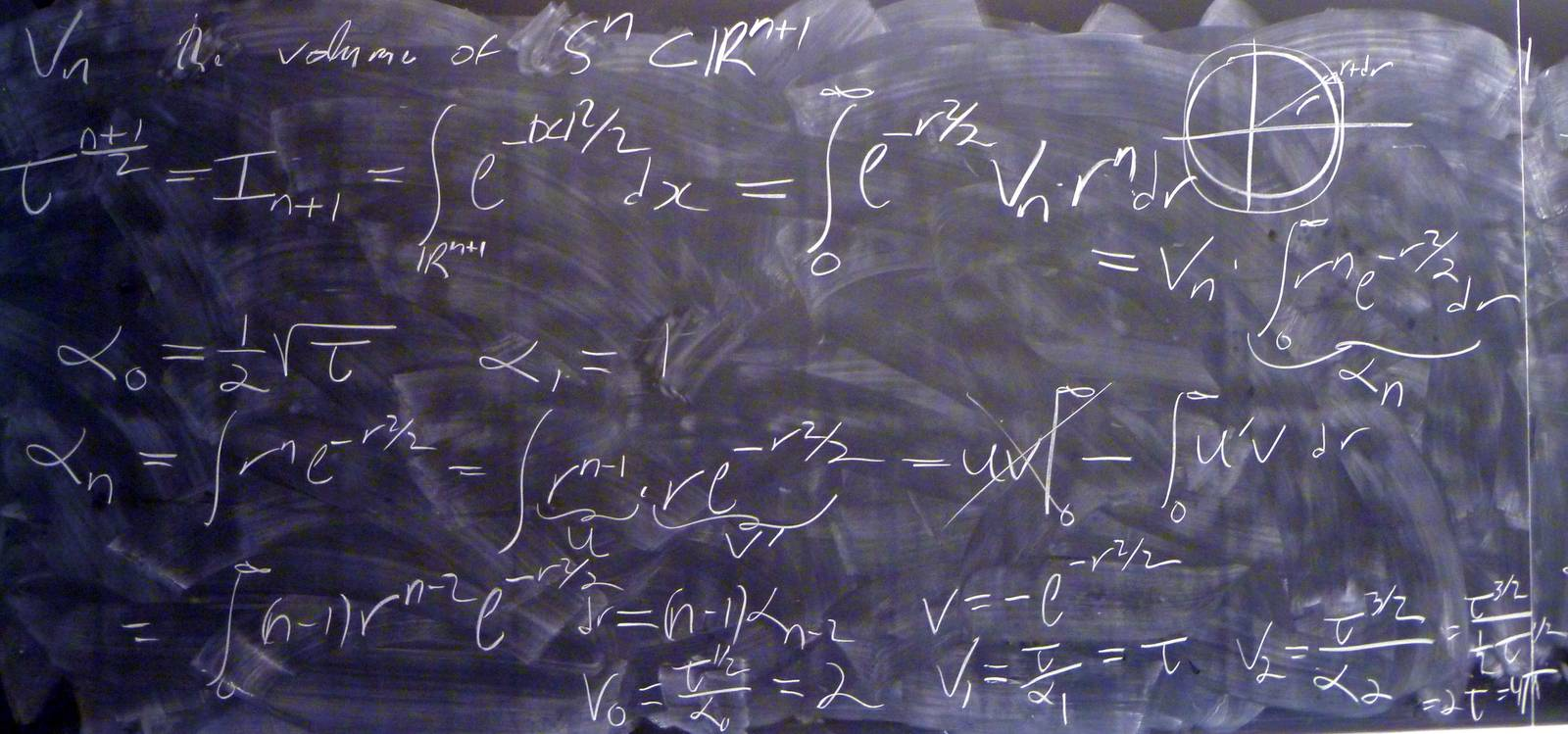© | Dror Bar-Natan: Classes: 2013-14: AKT: < >

# AKT-140117 Video

 width: 400 720 orig/AKT-140117.MOD For now, this video can only be viewed with web browsers that support

Notes on AKT-140117:    [edit, refresh]

Euler-Lagrange problems, Gaussian integration, volumes of spheres.

# Week of... Notes and Links
1 Jan 6 About This Class (PDF).
: Course introduction, knots and Reidemeister moves, knot colourings.
Tricolourability without Diagrams
: The Gauss linking number combinatorially and as an integral.
: The Schroedinger equation and path integrals.
Friday Introduction (the quantum pendulum)
2 Jan 13 Homework Assignment 1.
: The Kauffman bracket and the Jones polynomial.
: Self-linking using swaddling.
: Euler-Lagrange problems, Gaussian integration, volumes of spheres.
3 Jan 20 Homework Assignment 2.
: The definition of finite-type and some examples.
: The self-linking number and framings.
: Integrating a polynomial times a Gaussian.
Class Photo.
4 Jan 27 Homework Assignment 3.
: Chord diagrams and weight systems.
: Swaddling maps and framings, general configuration space integrals.
: Some analysis of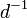$d^{-1}$.
5 Feb 3 Homework Assignment 4.
: 4T, the Fundamental Theorem and universal finite type invariants.
The Fulton-MacPherson Compactification (PDF).
: The Fulton-MacPherson Compactification, Part I.
: More on pushforwards,$d^{-1}$, and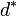$d^\ast$.
6 Feb 10 Homework Assignment 5.
: The bracket-rise theorem and the invariance principle.
: The Fulton-MacPherson Compactification, Part II.
: Gauge fixing, the beginning of Feynman diagrams.
R Feb 17 Reading Week.
7 Feb 24 : A review of Lie algebras.
: Graph cohomology and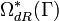$\Omega_{dR}^\ast(\Gamma)$.
: More on Feynman diagrams, beginning of gauge theory.
From Gaussian Integration to Feynman Diagrams (PDF).
8 Mar 3 Homework Assignment 6 (PDF)
: Lie algebraic weight systems.
: Graph cohomology and the construction of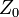$Z_0$.
Graph Cohomology and Configuration Space Integrals (PDF)
: Gauge invariance, Chern-Simons, holonomies.
Mar 9 is the last day to drop this class.
9 Mar 10 Homework Assignment 7 (PDF)
: The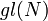$gl(N)$ weight system.
: The universal property, hidden faces.
: Insolubility of the quintic, naive expectations for CS perturbation theory.
10 Mar 17 Homework Assignment 8 (PDF)
: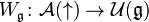$W_{\mathfrak g}\colon{\mathcal A}(\uparrow)\to{\mathcal U}({\mathfrak g})$ and PBW.
: The anomaly.
: Faddeev-Popov, part I.
Gaussian Integration, Determinants, Feynman Diagrams (PDF).
11 Mar 24 Homework Assignment 9 (PDF)
: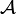${\mathcal A}$ is a bi-algebra.
: Understanding and fixing the anomaly.
Friday: class cancelled.
12 Mar 31 Monday, Wednesday: class cancelled.
: A Monday class: back to expansions.
E Apr 7 : A Friday class on what we mostly didn't have time to do.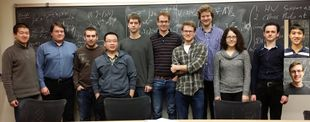Add your name / see who's in!
Dror's Notebookrefresh
panel
Managed by dbnvp: You can click on many of the blackboard shots / frame grabs to see their full-size versions.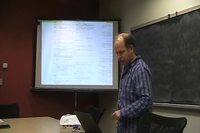0:02:49 [add] Handout view 2: The Question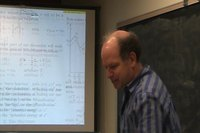0:03:36 [add] Handout view 3: e^H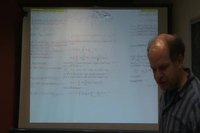0:04:11 [add] Handout view 4: The Trotter Formula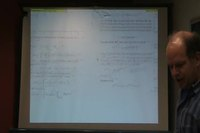0:04:28 [add] Handout view 5: Use Trotter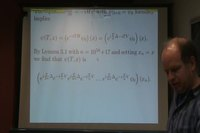0:04:43 [add] Handout view 6: e^V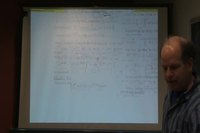0:04:55 [add] Handout view 7: e^Delta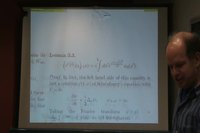0:05:10 [add] Handout view 8: Iterated convolutions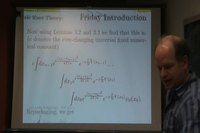0:05:37 [add] Handout view 9: A path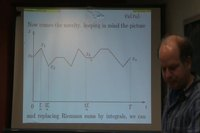0:06:04 [add] Handout view 10: Path integrals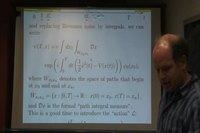0:06:45 [add] Handout view 11: The Action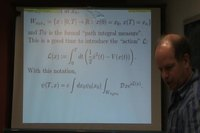0:07:19 [add] Handout view 12: Rid of Path Integrals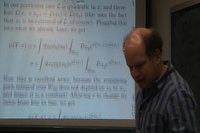0:10:56 [add] Handout view 13: Rid of PE, 2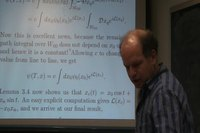0:11:00 [add] Handout view 14: Finding x_c0:16:43  The "power-line" problem is also known as the "catenary" problem (https://en.wikipedia.org/wiki/Catenary). As stated, we want to minimize the potential energy, so we must find an expression for the total potential energy of the system, which will be done by integrating along the rope/wire/line. The infinitesimal potential energy is given by $dV = gydm$, where g is the acceleration due to gravity, y is the height, and $dm$ is the infinitesimal mass along a length of the rope. In addition, $dm = \frac{m}{s}dl$, where $dl = \sqrt{(dx)^2 + (dy)^2} = dx\sqrt{1+(\frac{dy}{dx})^2}$ and $s$ is the total arclength of the rope. If we let the rope span a length from $x = -L$ to $x = L$, the total potential gravitational energy is $V(y) = \int_{-L}^{L}\frac{mg}{s}y(x)\sqrt{1+(y'(x))^2}dx$. This is what we want to minimize. As usual, let $f(\epsilon) = V(y_c + \epsilon y_q)$, where $y_q(0) = y_q(L) = 0$, and set $\frac{d}{d\epsilon}f(\epsilon)\mid_{\epsilon = 0}$. For convenience, use dot notation for derivatives, and compute to get

$\frac{d}{d\epsilon}f(\epsilon)\mid_{\epsilon = 0} = \frac{mg}{s}\int_{-L}^{L}y_q\sqrt{1+\dot{y}_q^2}+y_c(1+\dot{y}_c^2)^{\frac{-1}{2}(\dot{y}_c\dot{y}_q)}dx = 0$

Thus, $0 = \frac{mg}{s}(I_1 + I_2)$, where $I_1 = \int_{-L}^L y_q\sqrt{1+\dot{y}_c^2}dx$ and $I_2 = \int_{-L}^L y_c\dot{y}_c\dot{y}_q(1+\dot{y}_c^2)^{\frac{-1}{2}}dx$.

Integrating $I_2$ by parts with $u = y_c\dot{y}_c(1+\dot{y}_c^2)^{\frac{-1}{2}}$ and $dv = \dot{y}_q dx$, and applying boundary conditions $y_q(-L) = y_q(L) = 0$, we obtain

$I_2 = \int_{-L}^L y_q\big(y_c\dot{y}_c^2\ddot{y}_c(1+\dot{y}_c^2)^{\frac{-3}{2}} - (\dot{y}_c^2 + y_c\ddot{y}_c)(1+\dot{y}_c^2)^{\frac{-1}{2}})\big)dx$

From $I_1 + I_2 = 0$, factoring out the $y_q$ and the fundamental lemma of the calculus of variations, we obtain an ODE (replacing $y_c$ with $y$):

$0 = (1+\dot{y}^2)^\frac{-3}{2}((1+\dot{y}^2)^2 - (\dot{y}^2 + y\ddot{y})(1+\dot{y}^2) + y\dot{y}^2\ddot{y})$

Dividing through by $(1+\dot{y}^2)^\frac{-3}{2}$, expanding, and simplifying, we obtain our final ODE:

$1 + \dot{y}^2 - y\ddot{y} = 0$

A solution to this is $y(x) = \frac1{\lambda}cosh(\lambda x + c)$, where $\lambda$ and c are determined by physical constants and the boundary values of $y$ (at $x = -L$ and $x = L$). It turns out that this ODE is the same one you get when solving the soap bubble problem (HW 2, problem 3), since the Lagrangians of the two systems are the same up to constants.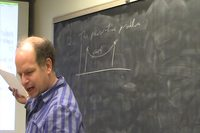0:16:59 [add] Handout view 13: Rid of PE, 2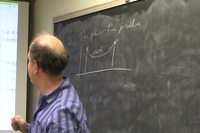0:17:10 [add] Handout view 14: Finding x_c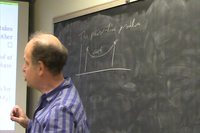0:17:15 [add] Handout view 13: Rid of PE, 2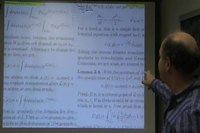0:17:18 [add] Handout view 14: Finding x_c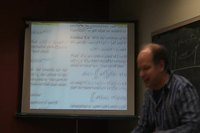0:17:38 [add] Handout view 14: Finding x_c
0:18:28 [add] The goal for 2-3 Fridays.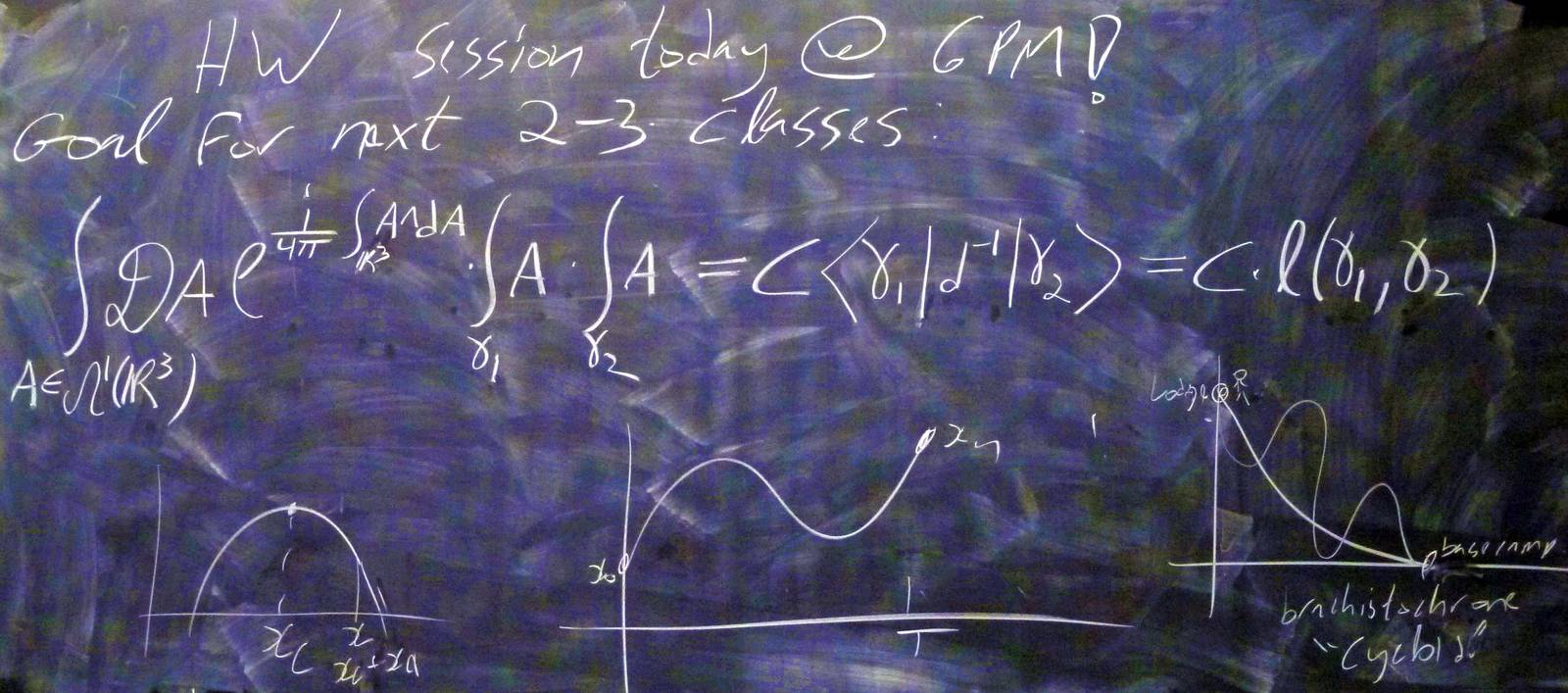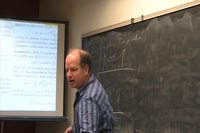0:18:40 [add] Handout view 15: Get Fourier!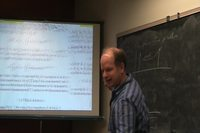0:18:43 [add] Handout view 14: Finding x_c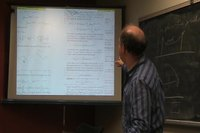0:19:46 [add] Handout view 11: The Action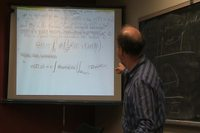0:19:48 [add] Handout view 12: Rid of Path Integrals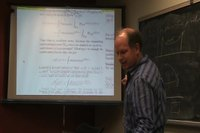0:19:53 [add] Handout view 14: Finding x_c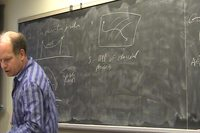0:21:05 [add] Handout view 15: Get Fourier!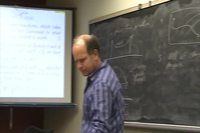0:21:09 [add] Handout view 17: E-L, 10:21:24  This is a more detailed derivation of the result from Lemma 3.4.

Let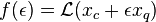$f(\epsilon) = \mathcal{L}(x_c + \epsilon x_q)$. This now becomes a single variable minimum/maximum problem. We set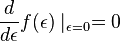$\frac{d}{d\epsilon}f(\epsilon)\mid_{\epsilon=0} = 0$, and solve for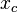$x_c$. First, simplifying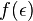$f(\epsilon)$, we compute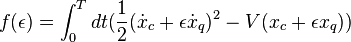$f(\epsilon) = \int_0^T dt(\frac{1}{2} (\dot{x}_c + \epsilon\dot{x}_q)^2 - V(x_c+\epsilon x_q))$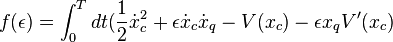$f(\epsilon) = \int_0^T dt(\frac{1}{2}\dot{x}_c^2 + \epsilon \dot{x}_c\dot{x}_q - V(x_c) - \epsilon x_q V'(x_c)$ + higher order terms).

Thus,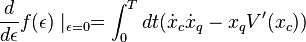$\frac{d}{d\epsilon}f(\epsilon)\mid_{\epsilon=0} = \int_0^T dt(\dot{x}_c\dot{x}_q - x_q V'(x_c))$

Integrating by parts with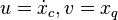$u = \dot{x}_c, v = x_q$, this is equal to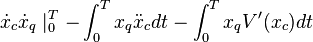$\dot{x}_c\dot{x}_q \mid_{0}^{T} - \int_0^T x_q\ddot{x}_c dt - \int_0^T x_q V'(x_c)dt$

The first term is equal to 0 by boundary conditions of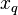$x_q$, so we obtain the equality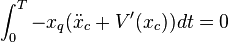$\int_0^T -x_q(\ddot{x}_c + V'(x_c))dt = 0$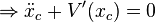$\Rightarrow \ddot{x}_c + V'(x_c) = 0$, exactly as stated in the conclusion of Lemma 3.4. Solving this ODE with initial conditions gives the desired result. Explicitly, the solution of this ODE (with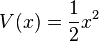$V(x) = \frac{1}{2}x^2$) is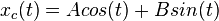$x_c(t) = Acos(t) + Bsin(t)$ Plugging in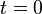$t = 0$ and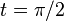$t = \pi/2$, we have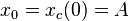$x_0 = x_c(0) = A$ and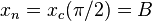$x_n = x_c(\pi/2) = B$, implying that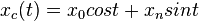$x_c(t) = x_0cos t + x_nsin t$, as claimed.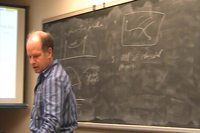0:25:14 [add] Handout view 18: E-L, 20:26:27  Just some generalization for the least action principle and Euler-Lagrange equation for the classical cases. In the calculus of variation, we have developed a tool for describing various physics situation. In general, let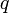$q$ be the coordinate of a particular configuration space and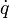$\dot{q}$ be its time derivative. Then, the action is described as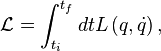$\mathcal{L}=\int_{t_i}^{t_f}dt L\left(q,\dot{q}\right),$

where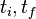$t_i,t_f$ are the initial time and final time, respectively. The integrand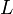$L$ is known as the Lagrangian and is assumed to be time-independent for convenience. The idea here is to find the path that minimize the action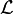$\mathcal{L}$. Now, we introduction the idea of variation, which can be viewed as an infinitesimal shift from the original path; however, it does not change the terminal points. Since the path we are interested is the path that minimizes the action, then the variation of the action should be 0 and that is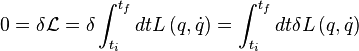$0=\delta \mathcal{L}=\delta \int_{t_i}^{t_f}dt L\left(q,\dot{q}\right)=\int_{t_i}^{t_f}dt \delta L\left(q,\dot{q}\right)$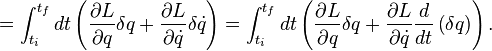$=\int_{t_i}^{t_f}dt \left(\frac{\partial L}{\partial q}\delta q+\frac{\partial L}{\partial\dot{q}}\delta \dot{q}\right)=\int_{t_i}^{t_f}dt \left(\frac{\partial L}{\partial q}\delta q+\frac{\partial L}{\partial\dot{q}}\frac{d}{dt}\left(\delta q\right)\right).$

Then, by the integration by parts, we have that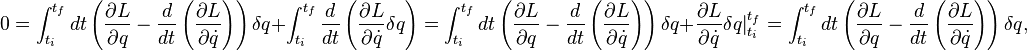$0=\int_{t_i}^{t_f}dt \left(\frac{\partial L}{\partial q}-\frac{d}{dt}\left(\frac{\partial L}{\partial\dot{q}}\right)\right)\delta q+\int_{t_i}^{t_f}\frac{d}{dt}\left(\frac{\partial L}{\partial\dot{q}}\delta q\right)=\int_{t_i}^{t_f}dt \left(\frac{\partial L}{\partial q}-\frac{d}{dt}\left(\frac{\partial L}{\partial\dot{q}}\right)\right)\delta q+\frac{\partial L}{\partial\dot{q}}\delta q\big|_{t_i}^{t_f}=\int_{t_i}^{t_f}dt \left(\frac{\partial L}{\partial q}-\frac{d}{dt}\left(\frac{\partial L}{\partial\dot{q}}\right)\right)\delta q,$

since the boundary term does not vary so that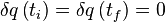$\delta q\left(t_i\right)=\delta q\left(t_f\right)=0$. Thus, we arrive at the point where the classical particle must obey the path where the equation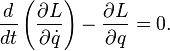$\frac{d}{dt}\left(\frac{\partial L}{\partial\dot{q}}\right)-\frac{\partial L}{\partial q}=0.$

This equation is known as the Euler-Lagrange equation.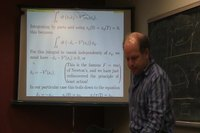0:27:05 [add] Handout view 19: E-L, 30:28:51  To demonstrate how to use the Euler-Lagrange equation in classical mechanics, we solve brachistochrone problem as an example. The problem is described in the blackboard shot, which is to find the path of a particle that minimizes the time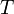$T$ traveled from point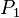$P_1$ to point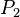$P_2$ in a uniform gravitational field. In this situation, we assume there is no friction along the path; thus the energy is conserved. Let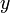$y$ be the vertical coordinate. Then, by the conservation of energy, we have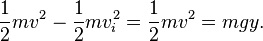$\frac{1}{2}mv^2-\frac{1}{2}mv_i^2=\frac{1}{2}mv^2=mgy.$

Thus, we have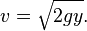$v=\sqrt{2gy}.$

Then, the time$T$ may be described as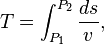$T=\int_{P_1}^{P_2}\frac{ds}{v},$

where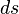$ds$ is the infinitesimal arclength of the path. Then, let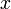$x$ be the horizontal coordinate, we have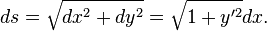$ds=\sqrt{dx^2+dy^2}=\sqrt{1+y'^2}dx.$ Thus, the above equation would be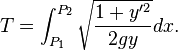$T=\int_{P_1}^{P_2}\sqrt{\frac{1+y'^2}{2gy}}dx.$

Now, let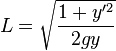$L=\sqrt{\frac{1+y'^2}{2gy}}$, we apply the Euler-Lagrange equation and obtain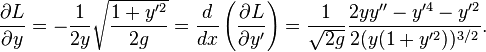$\frac{\partial L}{\partial y}=-\frac{1}{2y}\sqrt{\frac{1+y'^2}{2g}}=\frac{d}{dx}\left(\frac{\partial L}{\partial y'}\right)=\frac{1}{\sqrt{2g}}\frac{2yy''-y'^4-y'^2}{2(y(1+y'^2))^{3/2}}.$

If we rearrange the equation and integrate, we obtain the equation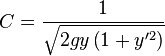$C=\frac{1}{\sqrt{2gy\left(1+y'^2\right)}}$

where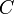$C$ is some constant. Then, we rearrange the equation and obatin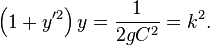$\left(1+y'^2\right)y=\frac{1}{2gC^2}=k^2.$

Then, we can solve this equation with parameterization and obtain the final result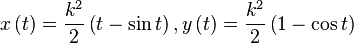$x\left(t\right)=\frac{k^2}{2}\left(t-\sin t\right),y\left(t\right)=\frac{k^2}{2}\left(1-\cos t\right)$

0:28:51 [add] Minimization problems.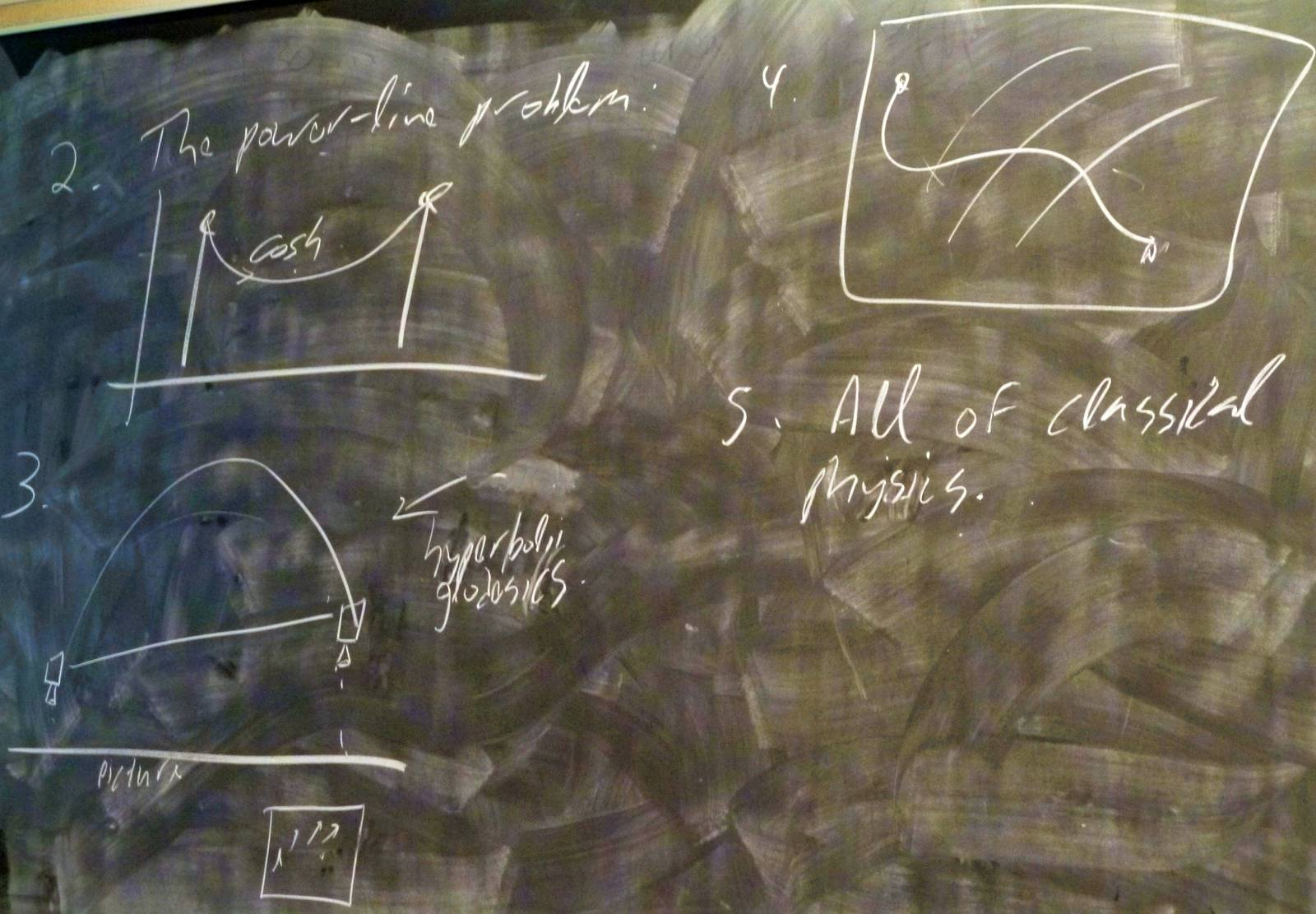0:39:51 [add] The most-basic Gaussian integration.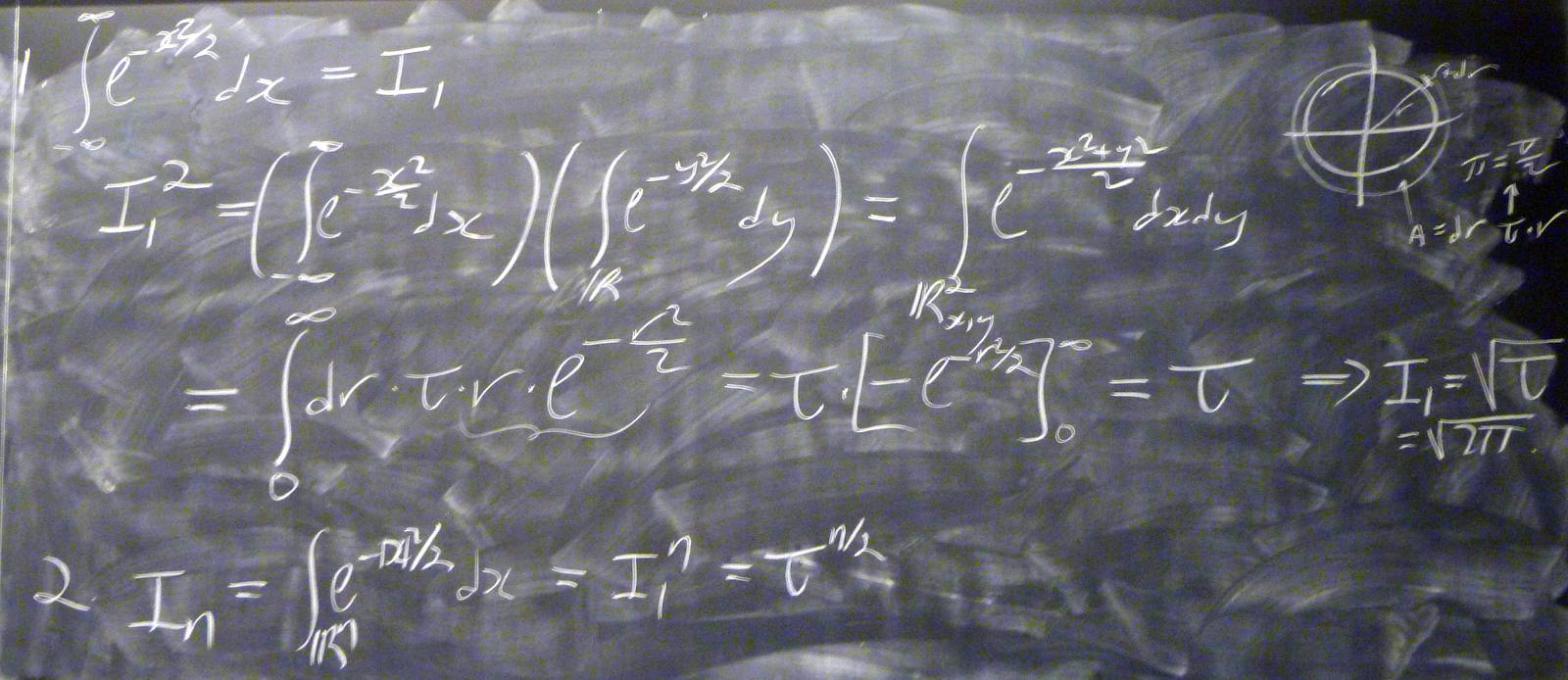0:51:02 [add] Volumes of spheres.